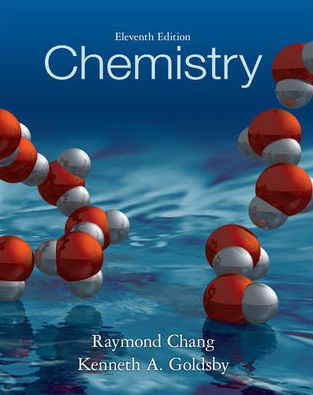×
×

# Solutions for Chapter 13: Chemistry 11th Edition## Full solutions for Chemistry | 11th Edition

ISBN: 9780073402680Solutions for Chapter 13

Solutions for Chapter 13
4 5 0 420 Reviews
13
1
##### ISBN: 9780073402680

Chemistry was written by and is associated to the ISBN: 9780073402680. This expansive textbook survival guide covers the following chapters and their solutions. Since 155 problems in chapter 13 have been answered, more than 317894 students have viewed full step-by-step solutions from this chapter. Chapter 13 includes 155 full step-by-step solutions. This textbook survival guide was created for the textbook: Chemistry, edition: 11.

Key Chemistry Terms and definitions covered in this textbook
• atmosphere (atm)

A unit of pressure equal to 760 torr; 1 atm = 101.325 kPa. (Section 10.2) atom The smallest representative particle of an element. (Sections 1.1 and 2.1)

A statement that the volume of a gas maintained at constant temperature and pressure is directly proportional to the number of moles of the gas. (Section 10.3)

• base

A substance that is an H+ acceptor; a base produces an excess of OH-1aq2 ions when it dissolves in water. (Section 4.3)

• Contributing structures

Representations of a molecule or ion that differ only in the distribution of valence electrons.

• crystal-field theory

A theory that accounts for the colors and the magnetic and other properties of transition-metal complexes in terms of the splitting of the energies of metal ion d orbitals by the electrostatic interaction with the ligands. (Section 23.6)

• curie

A measure of radioactivity: 1 curie = 3.7 * 1010 nuclear disintegrations per second. (Section 21.4)

• doping

Incorporation of a hetero atom into a solid to change its electrical properties. For example, incorporation of P into Si. (Section 12.7)

• enthalpy

A measure of the exchange of energy between the system and its surroundings during any process.

• Excited state

A state of a system at higher energy than the ground state.

• fuel value

The energy released when 1 g of a substance is combusted. (Section 5.8)

• Graham’s law

A law stating that the rate of effusion of a gas is inversely proportional to the square root of its molecular weight. (Section 10.8)

• Homotopic groups

Atoms or groups on an atom that give an achiral molecule when one of the groups is replaced by another group. The hydrogens of the CH2 group of propane, for example, are homotopic. Replacing either one of them with deuterium gives 2-deuteropropane, which is achiral. Homotopic groups have identical chemical shifts under all conditions

• joule (J)

The SI unit of energy, 1 kg@m2 >s2. A related unit is the calorie: 4.184 J = 1 cal. (Section 5.1)

• Mass spectrometry

An analytical technique for measuring the mass-to-charge ratio (m/z) of ions.

• meso compound

A compound that possesses chirality centers and an internal plane of symmetry.

• Phospholipid

A lipid containing glycerol esterifi ed with two molecules of fatty acid and one molecule of phosphoric acid.

• pi 1P2 molecular orbital

A molecular orbital that concentrates the electron density on opposite sides of an imaginary line that passes through the nuclei. (Section 9.8)

• reductive amination

The conversion of a ketone or aldehyde into an imine under conditions in which the imine is reduced as soon as it is formed, giving an amine.

• strong activators

Groups that strongly activate an aromatic ring toward electrophilic aromatic substitution, thereby significantly enhancing the rate of the reaction.

• Wolff-Kishner reduction

Reduction of the C"O group of an aldehyde or ketone to a CH2 group using hydrazine and a base. Ylide (Section 16.6)

×